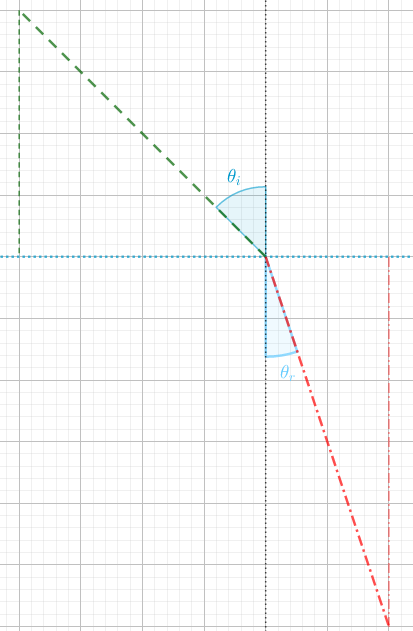### Math Notes

Subjects

#### Trigonometry Solutions

##### Topics || Problems

The speed of light in ethyl alcohol is approximately 220, 400 km/sec. A light ray leaves a point in the air and strikes a point in a jar of ethyl alcohol with an angle of refraction of 38 degrees. To the nearest minute, what is the angle of incidence?From Snell's Law $$\frac{sine ~ of ~angle ~ of~ incidence}{sine ~of~angle~of~refraction} \\= \frac{velocity~of~light~in~1^{st}~meduim}{velocity~of~ligth~in~2^{nd}~meduim}$$

The speed of light in air is approximately $$299,792 \frac{km}{sec}$$.

Thus, $$\frac{\sin \theta_i}{\sin \theta_r} = \frac{299,792}{220, 400}$$

$$\frac{\sin \theta_i}{\sin 38} = \frac{299,792}{220, 400}$$

$$\theta^{-1} = \sin ((\sin 38 )(1.36))$$

$$\theta^{-1} = 56^o 52'$$